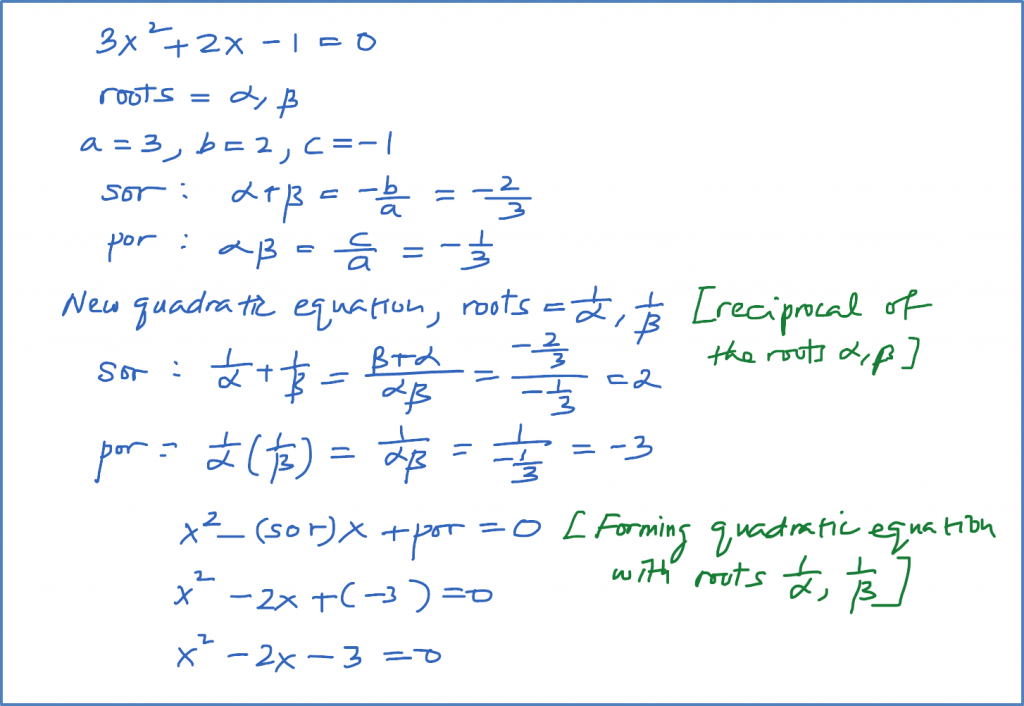\

# 2.12.2 Quadratic Functions, SPM Practice (Paper 1)

Question 4:
If the roots of find the equations whose roots are

Solution:Question 5:
Write and simplify the equation whose roots are double the roots of $3{x}^{2}-5x-1=0$ , without solving the given equation.

Solution:Question 6:
Write and simplify the equation whose roots are the reciprocals of the roots of $3{x}^{2}+2x-1=0$ , without solving the given equation.

Solution:### 1 thought on “2.12.2 Quadratic Functions, SPM Practice (Paper 1)”

1.Question 10:
At b^2 – 4ac > 0, should be 8p, instead of 4p.